Next: Estimation Up: Models Previous: Two-level GLM

## Single-level GLM

The two-level model written above can be re-written as a single-level model by substituting equation 2 into a block form of equation 1, giving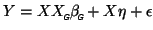(3)

where now all the first-level GLMs have been combined such that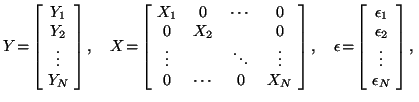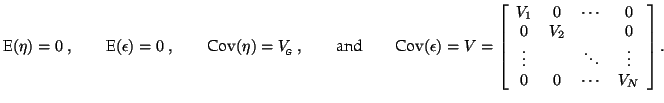The two error terms in equation 3 can simply be combined such that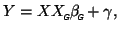(4)

where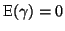and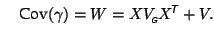It is easy to see that this model is equivalent to the two-level version presented as equations 1 and 2.

Christian Beckmann 2003-07-16